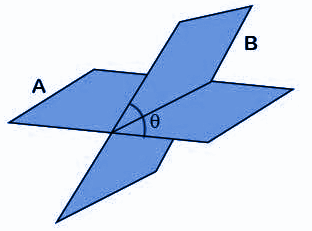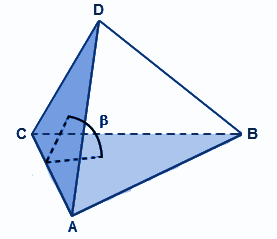# Dihedral Angle

Dihedral angle is defined when two planes intersect each other. The two intersecting planes here are the cartesian planes. The cartesian geometry is defined for two-dimensional and three-dimensional planes, which determines the shapes of different objects.

In general, we usually a combination of two lines or line segments to represent the angles. But in Geometry, we deal with the behaviour of two or more planes together. The problems related to two or more planes can be seen very often. The planes can also intersect one another. When we talk about the three-dimensional system, given two distinct planes either intersect each other or are parallel to each other. When two planes intersect, they are inclined at some angle which is known as the dihedral angle. In this article, we will discuss its definition, formula, method of its calculation and some solved examples.

## Dihedral Angle Definition

In three dimensional geometry, two planes can directly or indirectly (by extension) intersect each other. The angle formed between the intersection of two different planes is called a dihedral angle. In other words, we can say that the interior angle between the two planes is known as a dihedral angle.From the above figure, we can see, the two planes A and B are intersecting each other with an inclination of angle θ.

Points to Remember:

• As we can see that usually there are two angles formed between two planes – one is acute, and another is obtuse. Generally, the dihedral angle refers to the acute angle between the two planes. The obtuse angle can be found by subtracting an acute angle from 180°.
• Instead of intersecting two planes, sometimes they are parallel to each other. In this case, the dihedral angle between the two planes is zero. Hence, to prove that two planes are parallel, calculating the dihedral angle can be very useful.
• The dihedral angle can also be referred to as the interior angle made by the two planes in any three-dimensional shape, such as polyhedron. In the diagram, below β represents the dihedral angle between the two faces of a tetrahedron.## Dihedral Angle Formula

The dihedral angle is the angle between the normal vectors of two planes. Let us recall that the normal vector is defined as a vector that is perpendicular or normal or perpendicular to the given plane. Let us suppose that a plane is denoted by the equation :

px + qy + rz + s = 0 and its normal vector is represented by $$\vec{n}$$, then

$$\vec{n}$$ =(p, q, r)

Assume that the equations of two planes are given as:

p1x + q1y + r1z + s1 = 0 and p2x + q2y + r2z + s2 = 0

Let the corresponding normal vector to these planes be $$\vec{n_{1}}$$ and $$\vec{n_{2}}$$ respectively. Then:

$$\vec{n_{1}}$$ = (p1, q1, r1) and $$\vec{n_{2}}$$ = (p2, q2, r2)

If the acute angle between these planes be θ, then the formula for α would be given as below :

$$cos \theta = \frac{\vec{n_{1}}. \vec{n_{2}}}{\vec{n_{1}}\vec{n_{2}}}$$

It can also be written as:

$$cos \theta = \frac{|p_{1}p_{2} + q_{1}q_{2} + r_{1}r_{2}|}{\sqrt{p_{1}^{2}+ q_{1}^{2}+r_{1}^{2}} \sqrt{p_{2}^{2}+ q_{2}^{2}+r_{2}^{2}}}$$

### How to Calculate the Dihedral Angle?

To calculate the value of the dihedral angle, follow the below steps:

Step 1: Determine the equations of the plane and rearrange the terms in the following format: px + qy + rz + s = 0.

Step 2: Now, write the normal vector for each given plane, i.e. (p, q, r).

Step 3: Plug above values in the formula of dihedral angle which is:

$$cos \theta = \frac{|p_{1}p_{2} + q_{1}q_{2} + r_{1}r_{2}|}{\sqrt{p_{1}^{2}+ q_{1}^{2}+r_{1}^{2}} \sqrt{p_{2}^{2}+ q_{2}^{2}+r_{2}^{2}}}$$

Step 4: Solve and calculate the value of θ.

### Dihedral Angle Example

Question: Calculate the angle between two planes: x + 4y + z = 0 and 3 x + y + 4z = 0

Solution:

Given two planes: x + 4y + z = 0 and 3 x + y + 4z = 0

Compare the given plane equation with the standard form:

p1x + q1y + r1z + s1 = 0 and p2x + q2y + r2z + s2 = 0

So, we get :

p1 = 1, q1 = 4, r1 = 1

p2 = 3, q2 = 1, r2 = 4

The formula to find the dihedral angle is

$$cos \theta = \frac{|p_{1}p_{2} + q_{1}q_{2} + r_{1}r_{2}|}{\sqrt{p_{1}^{2}+ q_{1}^{2}+r_{1}^{2}} \sqrt{p_{2}^{2}+ q_{2}^{2}+r_{2}^{2}}}$$

$$cos \theta = \frac{|1.3 +4.1 + 1.4|}{\sqrt{1+ 16+1} \sqrt{9+ 1+16}}$$

$$cos \theta = \frac{|11|}{\sqrt{18} \sqrt{26}}$$

$$cos \theta = \frac{|11|}{\sqrt{468}} = 0.5085$$

Stay tuned with BYJU’S – The Learning App and also download the app to learn all the important Maths-related articles and explore videos.